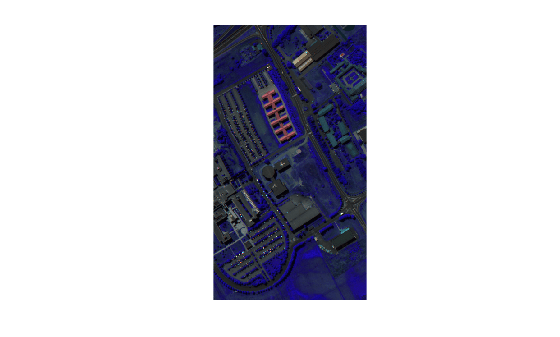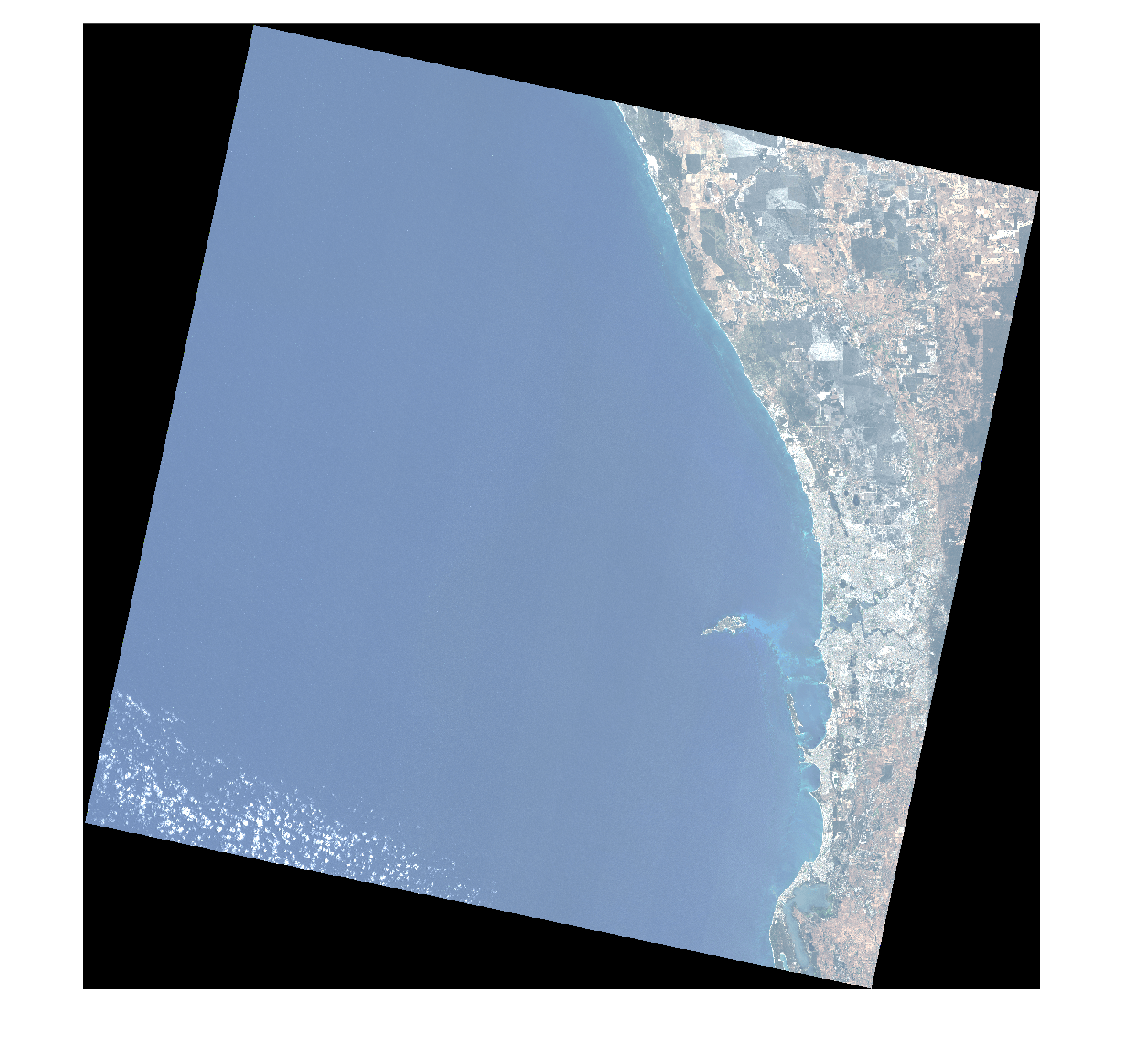# colorize

Estimate color image of hyperspectral data

## Syntax

``coloredImage = colorize(hcube)``
``coloredImage = colorize(hcube,band)``
``[coloredImage,indices] = colorize(___)``
``___ = colorize(___,Name,Value)``

## Description

example

````coloredImage = colorize(hcube)` estimates a false-color image of hyperspectral data based on the three most informative bands of the `hypercube` object `hcube`.```
````coloredImage = colorize(hcube,band)` returns a false-color image using the specified spectral bands `band`.```

example

````[coloredImage,indices] = colorize(___)` returns the indices of the bands used in the color image.```
````___ = colorize(___,Name,Value)` specifies options using one or more name-value pair arguments in addition to any combination of arguments from previous syntaxes. Use this syntax to specify the options to estimate false-colored and color-infrared (CIR) images of the input data. NoteThis function requires the Image Processing Toolbox™ Hyperspectral Imaging Library. You can install the Image Processing Toolbox Hyperspectral Imaging Library from Add-On Explorer. For more information about installing add-ons, see Get and Manage Add-Ons. ```

## Examples

collapse all

Read a hyperspectral data into the workspace.

`hcube = hypercube('paviaU.dat');`

Estimate a false-color image of the hyperspectral data.

`coloredImg = colorize(hcube);`

Display the false-color image.

`imshow(coloredImg)`Read a hyperspectral data into the workspace.

`hcube = hypercube('paviaU.dat');`

Estimate an RGB image of the hyperspectral data. Increase the image contrast by applying contrast stretching.

`coloredImg = colorize(hcube,"Method","rgb","ContrastStretching",true);`

Display the contrast-stretched RGB image.

`imshow(coloredImg)`## Input Arguments

collapse all

Input hyperspectral data, specified as a `hypercube` object. The `DataCube` property of the `hypercube` object stores the hyperspectral data cube as an M-by-N-by-C numeric array, where C is the number of bands.

Spectral band numbers, specified as a 3-element vector of positive integers. All elements of the vector must be less than or equal to the total number of bands C in the input data.

Data Types: `double`

### Name-Value Arguments

Specify optional pairs of arguments as `Name1=Value1,...,NameN=ValueN`, where `Name` is the argument name and `Value` is the corresponding value. Name-value arguments must appear after other arguments, but the order of the pairs does not matter.

Before R2021a, use commas to separate each name and value, and enclose `Name` in quotes.

Example: `colorize(hcube,'Method','rgb')`

Method used to visualize the bands, specified as the comma-separated pair consisting of `'Method'` and one of these options.

• `'falsecolored'` — Create a false-color image consisting of the three most informative bands selected using `selectBands` function.

• `'rgb'` — Create an RGB image by dividing the spectral range into red (R), green (G), and blue (B) bands. The red band ranges from 600 nm to 700 nm, the green band ranges from 500 nm to 600 nm, and the blue band ranges from 400 nm to 500 nm. The displayed R, G, and B channels consist of the most representative bands within the corresponding spectral range based on the correlation coefficient metric.

• `'cir'` — Create a color-infrared (CIR) image by dividing the spectral range into near infrared (NIR), R, and G bands. The NIR band ranges from 760 nm to 960 nm, the red band ranges from 600 nm to 700 nm, and the green band ranges from 500 nm to 600 nm. The displayed channels consist of the most representative bands within the corresponding spectral range based on the correlation coefficient metric.

To create RGB or CIR images, the `Wavelength` property of the `hypercube` object `hcube` must have wavelengths in each of the corresponding ranges.

Data Types: `char` | `string`

Perform contrast stretching of the image, specified as the comma-separated pair consisting of `'ContrastStretching'` and the logical `0` (`false`) or `1` (`true`). When `true`, the `colorize` function applies contrast-limited adaptive histogram equalization, using the `adapthisteq` function.

Data Types: `logical`

## Output Arguments

collapse all

Color image, returned as an M-by-N-by-3 numeric array. Each of the three color planes contains one band of the hyperspectral image.

Data Types: `single` | `double`

Indices of the selected bands, returned as a 3-element column vector of positive integers.

Data Types: `double`

## Version History

Introduced in R2020a